# Centre

(diff) ← Older revision | Latest revision (diff) | Newer revision → (diff)

A kind of pattern of the trajectories of an autonomous system of ordinary second-order differential equations (*) in a neighbourhood of a singular point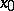, where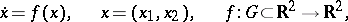(*)andis a domain of uniqueness. This kind of pattern is characterized as follows. There exists a neighbourhood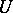ofsuch that all trajectories of the system starting in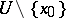are closed curves going around. Here the pointitself is also called a centre. In the figure the point 0 is the centre. The motion along trajectories with increasingcan proceed clockwise (indicated in the figure by arrows) or counterclockwise. A centre is Lyapunov stable (but not asymptotically stable). Its Poincaré index is 1.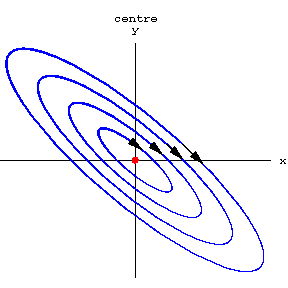Figure: c021230a

A pointis a centre for a system (*), for example when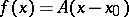, whereis a constant matrix with pure imaginary eigen values. In contrast to simple rest points of other types that occur for linear second-order systems (a saddle, a node or a focus), a pointof centre-type does not, generally speaking, remain a centre under a perturbation of the right-hand side of the linear system, whatever the order of smallness of the perturbations relative toand the order of their smoothness may be. It can then change into a focus (stable or unstable) or into a centre-focus (see Centre and focus problem). For a non-linear system (*) of class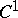(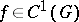) a rest pointcan be a centre also in the case when the matrix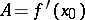has two zero eigen values.